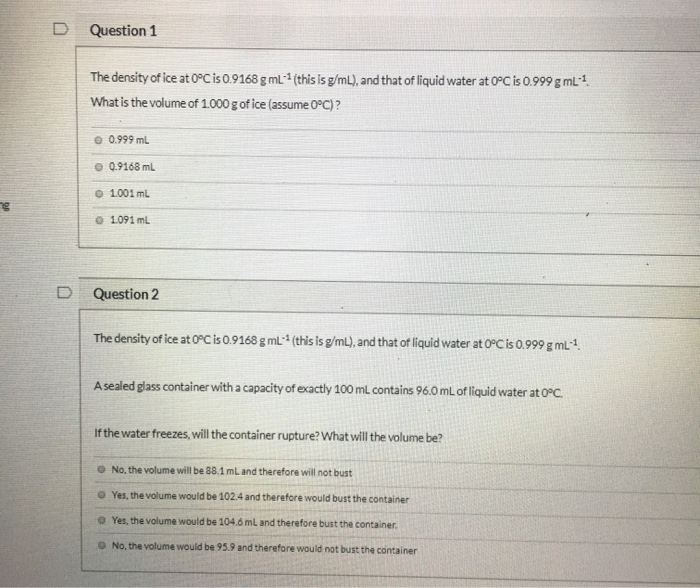# Solved: Question 1 The Density Of Ice At 0°C Is 0.9168 G ML:1 (this Is G/mL), And That Of Liquid Water At 0C Is 0.999 G ML:1. What Is The Volume Of 1000 G Of Ice (assume 0C)? 0.999 ML O 0.9168 ML O 1.

By |Question 1 The density of ice at 0°C is 0.9168 g mL:1 (this is g/mL), and that of liquid water at 0C is 0.999 g mL:1. What is the volume of 1000 g of ice (assume 0C)? 0.999 mL O 0.9168 mL o 1.001 mL o 1.091 mL D Question 2 The density of ice at 0°C is 0.9168 g mL:1 (this is g/mL), and that of liquid water at 0PC is 0.999 g mL*1. Asealed glass container with a capacity of exactly 100 mL contains 96.0 mLof liquid water at 0PC If the water freezes, will the container rupture? What will the volume be? O No. the volume will be 88.1 mL and therefore will not bust O Yes, the volume would be 102.4 and therefore would bust the container O Yes, the volume would be 104.6 mL and therefore bust the container O No, the volume would be 95.9 and therefore would not bust the container